# Nonlinear Filters

The Nonlinear Filters are not weighted averages of the neighboring input grid values; however, they are simple functions of the neighboring input grid values.

## Order Statistics Filters

 Minimum (mxn) The output grid node value equals the minimum of the neighboring values. Lower Quartile (mxn) The output grid node value equals the twenty-five percentile of the neighboring values. Median (mxn) The output grid node value equals the fifty percentile of the neighboring values. Upper Quartile (mxn) The output grid node value equals the seventy-five percentile of the neighboring values. Maximum (mxn) The output grid node value equals the maximum of the neighboring values. Range (mxn) The output grid node value equals the maximum minus the minimum of the neighboring values.

## Moment Statistics Filters

 Standard Deviation (mxn) The output grid node value equals the standard deviation of the neighboring values. The standard deviation is the square root of the variance. Variance (mxn) The output grid node value equals the variance of the neighboring values. The computation involves three steps. First, count the number of non-NoData (i.e. non-blank) input grid node values in the neighborhood. With a neighborhood height S and width T, compute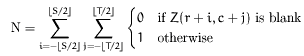Second calculate the local average,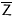,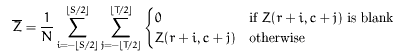Third, compute the local variance as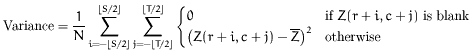Coef. of Variation (mxn) The output grid node value equals the coefficient of variation of the neighboring values. The coefficient of variation is the standard deviation divided by the average.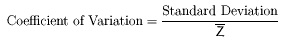If the average (the denominator) is too small, then the coefficient of variation is arbitrarily set equal to 0.

## Other Nonlinear Filters

 Median Difference (mxn) For each output grid node, (r, c), identify the set of non-NoData (non-blank), neighboring input grid node values. Compute the median of these neighboring values. Let B represent this median value. Then the output grid node value is set equal to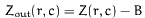The effect of this filter is to emphasize outliers in the grid. Threshold Averaging (mxn) For each output grid node, (r, c), identify the set of non-NoData (non-blank), neighboring input grid node values. Compute the average of these neighboring values, excluding the value of the corresponding input grid node. Let A represent this average value. Then the output grid node value is set equal to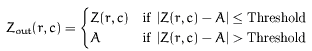where Threshold is the specified value. The effect of this filter is to eliminate outliers in the grid.